# Olympiad Test: Motion And Time

## 15 Questions MCQ Test Science Olympiad Class 7 | Olympiad Test: Motion And Time

Description
Attempt Olympiad Test: Motion And Time | 15 questions in 30 minutes | Mock test for Class 7 preparation | Free important questions MCQ to study Science Olympiad Class 7 for Class 7 Exam | Download free PDF with solutions
QUESTION: 1

Solution:
QUESTION: 2

Solution:
QUESTION: 3

### One mean solar day is equal to how many seconds?

Solution:

Mean solar day = 24 h
= 24 × 60 × 60
= 86400 seconds

QUESTION: 4

Which of the following graphs does not represent constant speed?

Solution:

In a constant speed the change in position of the body remains the same for a particular interval of time. Break time axis into small parts and see the distance travelled in each of these parts is the same in choices A, B and C (zero in ‘C’). But the distance changes in ‘D’.

QUESTION: 5

Which of the following is not in uniform motion?

Solution:
QUESTION: 6

Which of the following does not show oscillatory motion?

Solution:
QUESTION: 7

A jet is moving with a speed of 180 km/h. What is its speed in m/s?

Solution: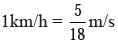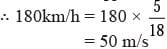QUESTION: 8

Which of the following is the fastest?

Solution:
QUESTION: 9

Which of the following is matched incorrectly?

Solution:

An odometer measures distances.

QUESTION: 10

Distance travelled will be equal to the displacement if

Solution:
QUESTION: 11

The area of the shaded portion in the graph shown below represents?​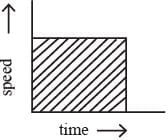Solution:
QUESTION: 12

The following graph shows the motion of four runners. P, Q, R and S in a 5 km marathon. Whose motion is the fastest?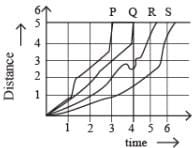Solution:

The time taken by P is the lowest among the four, because more is the area under the wine, greater is the speed. Hence, P is the fastest.

QUESTION: 13

With what speed should a car travel so that it can cover a distance of 5 km in 5 min?

Solution: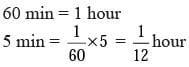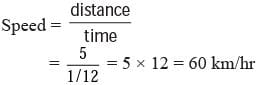QUESTION: 14

All of the following are units time except.

Solution:

A light year is the distance covered by light in one year, so it is the unit of distance and not of time.

QUESTION: 15

Study the graph given below. How is the speed of the moving body?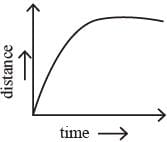Solution:

Speed is the change of position of the body with respect to time. From the graph it can be seen that the change becomes less with time till there is no change at all i.e. till the speed becomes zero.Use Code STAYHOME200 and get INR 200 additional OFF Use Coupon Code